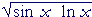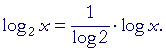Topics in

P R E C A L C U L U S

20

# LOGARITHMS

Definition

WHEN WE ARE GIVEN the base 2, for example, and exponent 3, then we can evaluate 23.

23 = 8.

Inversely, if we are given the base 2 and its power 8 --

2? = 8

-- then what is the exponent that will produce 8?

That exponent is called a logarithm.  We call the exponent 3 the logarithm of 8 with base 2.  We write

3 = log28.

We write the base 2 as a subscript.

3 is the exponent to which 2 must be raised to produce 8.

A logarithm is an exponent.

Since

104 = 10,000

then

log1010,000 = 4.

"The logarithm of 10,000 with base 10 is 4."

4 is the exponent to which 10 must be raised to produce 10,000.

"104 = 10,000" is called the exponential form.

"log1010,000 = 4" is called the logarithmic form.

Here is the definition:

logbx = n   means   bn = x.

That  base  with that  exponent  produces x.

Example 1.   Write in exponential form:   log232 = 5.

Answer.   25 = 32.

 Example 2.   Write in logarithmic form:  4−2  = 116 .
 Answer.   log4 116 = −2.

Problem 1.   Which numbers have negative logarithms?

To see the answer, pass your mouse over the colored area.
To cover the answer again, click "Refresh" ("Reload").
Do the problem yourself first!

Proper fractions.

Lesson 20 of Arithmetic

Example 3.   Evaluate  log81.

Answer.   8 to what exponent produces 1?  80 = 1.

log81 = 0.

We can observe that, in any base, the logarithm of 1 is 0.

logb1 = 0

Example 4.    Evaluate  log55.

Answer.   5 with what exponent will produce 5?   51 = 5.  Therefore,

log55 = 1.

In any base, the logarithm of the base itself is 1.

logbb = 1

log22m = ?

Answer.   2 raised to what exponent will produce 2m ?   m, obviously.

log22m = m.

The following is an important formal rule, valid for any base b:

logbbx = x

This rule embodies the very meaning of a logarithm.  x -- on the right -- is the exponent to which the base b must be raised  to produce bx.

The rule also shows that the exponential function bx is the inverse of the function logbx.  We will see this in the following Topic.

 Example 6 .   Evaluate  log3 19 .
 Answer. 19 is equal to 3 with what exponent? 19 = 3−2
 log3 19 = log33−2  =  −2.

Compare the previous rule.

Example 7.   log2 .25 = ?

Answer.   .25 = ¼ = 22.  Therefore,

log2 .25 = log22−2 = −2.

Example 8.   log3= ?

Answer.= 31/5.  (Definition of a rational exponent.)  Therefore,

log3= log331/5 = 1/5.

Problem 2.   Write each of the following in logarithmic form.

To see the answer, pass your mouse over the colored area.
To cover the answer again, click "Refresh" ("Reload").

 a)     bn = x logbx = n b)     23 = 8 log28 = 3 c)     102 = 100 log10100 = 2 d)     5−2 =  1/25. log51/25 = −2.

Problem 3.   Write each of the following in exponential form.

 a)   logbx = n bn = x b)   log232 = 5 25 = 32 c)   2 = log864 82 = 64 d)   log61/36 = −2 6−2 = 1/36

Problem 4.   Evaluate the following.

 a)   log216 = 4 b)   log416 = 2 c)   log5125 = 3 d)   log81 = 0 e)   log88 = 1 f)   log101 = 0

Problem 5.   What number is n?

 a)   log10n = 3 1000 b)   5 = log2n 32 c)   log2n = 0 1 d)   1 = log10n 10
 e)   logn 116 = −2 4 f)   logn 15 = −1 5 g)   log2 132 = n −5 h)   log2 12 = n −1

Problem 6.   logbbx  =  x

Problem 7.   Evaluate the following.

 a)   log9 19 = log99−1 = −1
 b)   log9 181 = −2 c)   log2 14 = −2 d)   log2 18 = −3 e)   log2 116 = −4
 f)   log10 .01 = −2 g)   log10 .001 = −3 h)   log6= 1/3 i)   logb= 3/4

Common logarithms

The system of common logarithms has 10 as its base.  When the base is not indicated,

log 100 = 2

then the system of common logarithms -- base 10 -- is implied.

Here are the powers of 10 and their logarithms:

 Powers of 10: 1   1000 1  100 110 1 10 100 1000 10,000 Logarithms: −3 −2 −1 0 1 2 3 4

Logarithms replace a geometric series with an arithmetic series.

Problem 8.   log 10n = ?   n.  The base is 10.

Problem 9.   log 58 = 1.7634.   Therefore, 101.7634 = ?

58.  1.7634 is the common logarithm of 58.  When 10 is raised to that exponent, 58 is produced.

Problem 10.   log (log x) = 1.  What number is x?

log a = 1, implies a = 10, which is the base.

Example 4.

Therefore, log (log x) = 1 implies log x = 10. Since 10 is the base,

x = 1010 = 10,000,000,000

Natural logarithms

The system of natural logarithms has the number called e as its base. (e is named after the 18th century Swiss mathematician, Leonhard Euler.)  e is the base used in calculus.  It is called the "natural" base because of certain technical considerations.

ex has the simplest derivative. Lesson 14 of An Approach to Calculus.)

e can be calculated from the following series expressed with factorials:

 e = 1 + 11! + 12! + 13! + 14! +  .   .  .

e is an irrational number; its decimal value is approximately

2.71828182845904.

To indicate the natural logarithm of a number we write "ln."

ln x  means  logex.

Problem 11.   What number is  ln e ?

ln e = 1.  The logarithm of the base itself is always 1.  e is the base.

Example 4.

Problem 12.   Write in exponential form (Example 1):   y = ln x.

ey = x.

e is the base.

The three laws of logarithms

1.    logbxy  =  logbx  +  logby

"The logarithm of a product is equal to the sum
of the logarithms of each factor.
"

 2.    logb=  logbx  −  logby

"The logarithm of a quotient is equal to the logarithm of the numerator
minus the logarithm of the denominator.
"

3.    logb xn =  n logbx

"The logarithm of x with a rational exponent is equal to
the exponent times the logarithm."

We will prove these laws below.

 Example 9.    Apply the laws of logarithms to  log abc2 d3 .

Answer.   According to the first two laws,

 log abc2 d 3 = log (abc2) − log d 3 = log a + log b + log c2 − log d 3 = log a + log b + 2 log c − 3 log d,

according to the third law.

The Answer above shows the complete theoretical steps.  In practice, however, it is not necessary to write the line

 log abc2 d 3 = log (abc2) − log d 3 .

The student should be able to go immediately to the next line --

 log abc2 d 3 = log a + log b + log c2 − log d 3

-- if not to the very last linelog abc2 d 3 = log a + log b + 2 log c − 3 log d.
 Example 10.   Apply the laws of logarithms to logz5 .
 Answer. logz5 =  log x  +  log−  log z5

Now,=  y½. (Lesson 29 of Algebra.)  Therefore, according to the third law:

 logz5 =  log x  +  ½ log y  −  5 log z.

Example 11.   Use the laws of logarithms to rewrite  ln.

Solution.

 ln= ln (sin x  ln x)½ = ½ ln (sin x  ln x),   3rd Law = ½ (ln sin x  +  ln ln x),   1st Law.

Note that the factors  sin x ln x  are the arguments of the logarithm function.

Example 12.   Solve this equation for x:

 log 32x + 5 = 1 Solution.   According to the 3rd Law, we may write (2x + 5)log 3 = 1. Now, log 3 is simply a number.  Therefore, on distributing log 3, 2x· log 3 + 5 log 3 = 1 2x· log 3 = 1 − 5 log 3 x = 1 − 5 log 3   2 log 3

By this technique, we can solve equations in which the unknown appears in the exponent.

Problem 13.   Use the laws of logarithms to rewrite the following.

 a)  log ab c = log a  +  log b  −  log c b)  log ab2 c4 = log a  +  2 log b  −  4 log c c)  logz = 1/3 log x  +  1/2 log y  −  log z

d)   ln (sin2x ln x = ln sin2x  +  ln ln x  =  2 ln sin x  +  ln ln x

 e)   ln=  ½ ln (cos x· x1/3 ln x) = ½ (ln cos x  +  1/3 ln x  +  ln ln x)
 f)   ln (a2x − 1 b5x + 1 ) =  ln a2x  −  1  +  ln b5x  +  1 = (2x − 1) ln a  +  (5x + 1) ln b

Problem 14.   Solve for x.

 ln 23x + 1 = 5. (3x + 1) ln 2 = 5 3x ln 2  +  ln 2 = 5 3x ln 2 = 5 − ln 2 x = 5 − ln 2  3 ln 2
 Problem 15.  Prove:    −ln x =  ln 1x .
 −ln x = (−1)ln x = ln x−1, Third law = ln 1x

Proof of the laws of logarithms

The laws of logarithms will be valid for any base.  We will prove them for base e, that is, for  y = ln x.

1.    ln ab  =  ln a  +  ln b.

The function  y = ln x  is defined for all positive real numbers x.  Therefore there are real numbers p and q such that

p = ln a   and   q = ln b.

This implies

a = e p   and   b = e q.

Therefore, according to the rules of exponents,

ab = e p· e q = e p + q.

And therefore

ln ab = ln e p + q = p + q = ln a + ln b.

Which is what we wanted to prove.

In a similar manner we can prove the 2nd law.  Here is the 3rd:

3.    ln an  =  n ln a.

There is a real number p such that

p = ln a;

that is,

a = e p.

And the rules of exponents are valid for all rational numbers n (Lesson 29 of Algebra; an irrational number is the limit of a sequence of rational numbers).  Therefore,

an = e pn.

This implies

ln an = ln e pn = pn = np = n ln a.

That is what we wanted to prove.

Change of base

Say that we know the values of logarithms of base 10, but not, for example, in base 2.  Then we can convert a logarithm in base 10 to one in base 2 -- or any other base -- by realizing that the values will be proportional.

Each value in base 2 will differ from the value in base 10 by the same constant k.

Now, to find that constant, we know thatTherefore, on putting x = 2 above:That impliesTherefore,That is,By knowing the values of logarithms in base 10, we can in this way calculate their values in base 2.

In general, then, if we know the values in base a, then the constant of proportionality in changing to base b, is the reciprocal of its log in base a.Problem 16.   Write the rule for changing to base 8 from base e.Next Topic:  Logarithmic and exponential functions

Please make a donation to keep TheMathPage online.
Even \$1 will help.